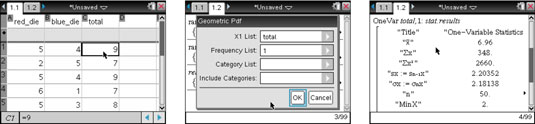##### TI-Nspire For Dummies, 2nd EditionData contained within the TI-Nspire Lists & Spreadsheet application can be analyzed from within the Calculator application. For example, you might be interested in viewing the one-variable statistical results of the Total list from the two-dice sum experiment. Follow these steps:

2. Press [ENTER] to indicate that you want to analyze one list.

If you have additional lists, change Num of Lists to match the number of lists you’re interested in analyzing.

3. Configure the dialog box by choosing X1 list total, as shown in the second screen.

4. Press [ENTER] to close the dialog box and perform the statistical analysis. (See the third screen.)A variety of statistical results are generated by the One-Variable Statistics command.

Many of the statistical results generated by the One-Variable Statistics command can be opened individually from within the List Math submenu (press [MENU]→Statistics→List Math). For example, the command Sum of Elements (denoted Sum) yields the sum of all the elements of a specified list. In general, all the commands found within the List Math submenu must contain either a list or a list name as their arguments.

You can sort lists or perform a variety of other manipulations of lists from within the Calculator application. Many of the commands that allow you to perform such manipulations are found in the Lists Operations submenu (press [MENU]→Statistics→List Operations). For example, the command Sort Ascending (denoted SortA) sorts a specified list from lowest value to highest value.